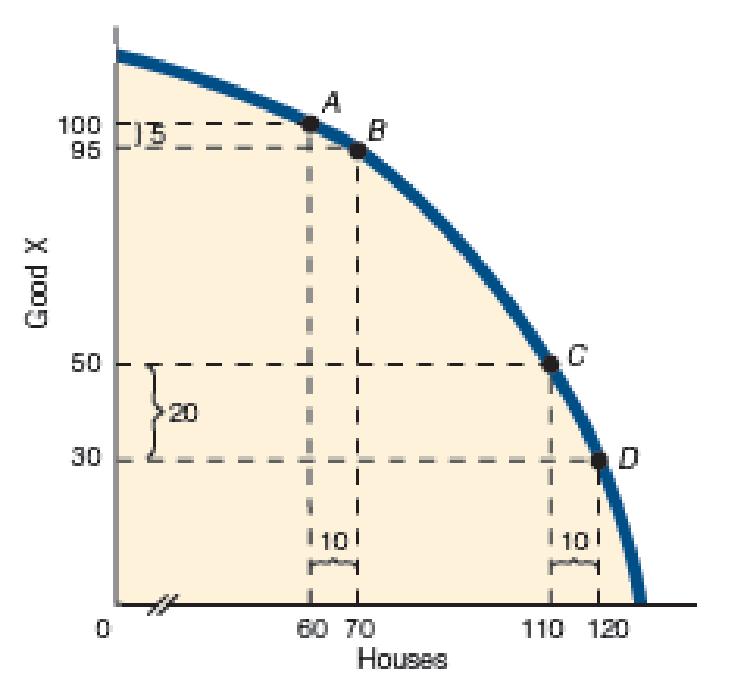Chapter 2, Problem 4QP

Chapter
Section
Textbook Problem

Look back at Exhibit 4 and notice that the slope between points A and B is relatively flatter than it is between points C and D. What does the slope of a curve between two points have to do with the opportunity cost of producing additional units of a good?To determine

Calculation of slope.

Explanation

Figure 1 shows the PPF curve of good X and houses.

In figure 1, vertical axis measures the good X and horizontal axis measures houses. The concave curve is the PPF (production possibility frontier).

The general formula for calculating slope is given below.

Slope=Cost of good Xpresent Costof good XpreviousQuantity of housespresent Quantity housesprevious        (1)

Substitute the respective values in equation (1) to calculation the slope of AB

Still sussing out bartleby?

Check out a sample textbook solution.

See a sample solution

The Solution to Your Study Problems

Bartleby provides explanations to thousands of textbook problems written by our experts, many with advanced degrees!

Get Started

Why are some economists against a target of zero inflation?

Brief Principles of Macroeconomics (MindTap Course List)

What are the key factors on which external financing depends, as indicated in the AFN equation?

Fundamentals of Financial Management, Concise Edition (with Thomson ONE - Business School Edition, 1 term (6 months) Printed Access Card) (MindTap Course List)

Why do executives and managers spend more time listening than do workers?

Essentials of Business Communication (MindTap Course List)